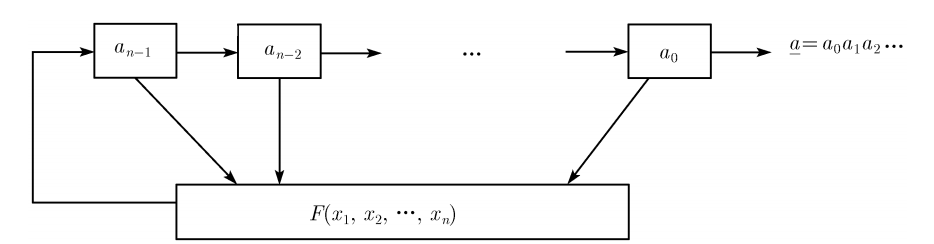# 反馈移位寄存器¶

Warning

The current page still doesn't have a translation for this language.

Besides, you can also help to translate it: Contributing.• $a_0$$a_1$，…，$a_{n-1}$，为初态。
• F 为反馈函数或者反馈逻辑。如果 F 为线性函数，那么我们称其为线性反馈移位寄存器（LFSR），否则我们称其为非线性反馈移位寄存器（NFSR）。
• $a_{i+n}=F(a_i,a_{i+1},...,a_{i+n-1})$

$(a_i,a_{i+1},...,a_{i+n-1}) \rightarrow (a_{i+1},...,a_{i+n-1},a_{i+n})$ . 对于一个序列来说，我们一般定义其生成函数为其序列对应的幂级数的和。